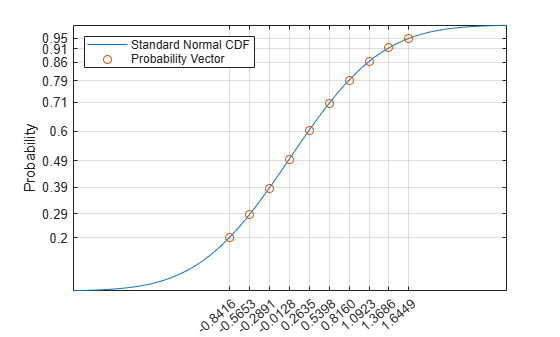# probgrid

Nonuniformly spaced probabilities

## Syntax

``p = probgrid(p1,p2)``
``p = probgrid(p1,p2,n)``

## Description

example

````p = probgrid(p1,p2)` returns a nonuniformly spaced array of 100 probabilities between `p1` and `p2` that correspond to the values of the normal cumulative distribution function (CDF) evaluated over a set of points uniformly spaced in the domain of the normal distribution.```

example

````p = probgrid(p1,p2,n)` returns an array of `n` probabilities.```

## Examples

collapse all

Evaluate the standard normal cumulative distribution function (CDF) on a 10-point grid between 0.2 and 0.95. Determine the points that correspond to the probabilities by evaluating the inverse normal CDF, also known as the probit function.

```pmin = 0.2; pmax = 0.95; N = 10; pd = probgrid(pmin,pmax,N); xd = sqrt(2)*erfinv(2*pd-1);```

Plot the standard normal CDF and overlay the points generated by `probgrid`.

```x = -3:0.01:3; sncdf = (1+erf(x/sqrt(2)))/2; plot(x,sncdf) hold on plot(xd,pd,'o') hold off legend({'Standard Normal CDF','Probability Vector'}, ... 'Location','Northwest') xticks(xd) xtickangle(40) yticks(round(100*pd)/100) ylabel('Probability') grid on```## Input Arguments

collapse all

Interval endpoints, specified as scalars from the interval [0, 1]. `p1` and `p2` must obey `p1` < `p2`.

Data Types: `double`

Number of samples in probability grid, specified as a positive integer scalar.

Data Types: `double`

## Output Arguments

collapse all

Array of probabilities, returned as a row vector.

## Version History

Introduced in R2021a